# National Aeronautics and Space Administration

## Goddard Space Flight Center# Using Newton's Law of Universal Gravitation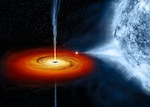Recap: Your astronomy professor has tasked the class with determining the mass of the black hole candidate, Cygnus X-1. You thought of three possible ways to do this, one of which will give you the right answer. You've decided to try using Newton's law of universal gravitation directly.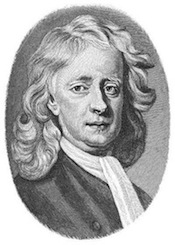Isaac Newton (Courtesy of the University of Texas Libraries, The University of Texas at Austin)

You know from the work you've been doing in astronomy class that the mass of Cygnus X-1 could be determined if a probe is launched toward it and programmed to orbit at a fixed distance. The probe would be kept in orbit around Cyg X-1 by the force of gravity, whose strength is dependent only upon Cyg X-1's mass. The probe could then radio back information about its orbital speed, and you would be able to determine the mass of Cyg X-1 with that information.

It would be a simple matter of using Newton's law of universal gravitation and the equations for circular motion.

How would this work mathematically? You realize that if you balance the forces on the probe, you can get the mass. First look at the probe and the forces acting on it. The gravitational attraction of Cygnus X-1 pulls the probe inward, toward the black hole. The centripetal force of the circular motion of the probe pushes it outward, and the two are equally balanced.Illustration of a probe orbiting Cygnus X-1 and the forces acting on the probe.(Credit: NASA's Imagine the Universe)

So, you take the two forces...

...and equate them:

Where G is Newton's gravitational constant, M is the mass of Cyg X-1, m is the mass of the probe, r is the distance of the probe from the center of Cyg X-1, and v is the orbital velocity of the probe.

Because m, the mass of the satellite, appears on both sides of the equation, it can be eliminated. Rearranging to solve for M, you get an expression with the measurable values of the probe's speed and distance from Cyg X-1 and the known value of Newton's constant of universal gravitation, G.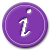Learn more about Newton's law of universal gravitationLearn more about uniform circular motion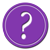Try using this technique to find the Sun's mass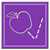Use this technique to solve for Cyg X-1's mass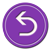Return to the beginning and try another approach